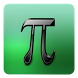# Math Pieces - Problem SolverFree App

Rated 0.00/5 (0) —  Free Android application by Enjoy & Learn Games

## About Math Pieces - Problem Solver

Now you have all useful math formulas and definitions handy and can always refer to them. , explaining all symbols used in formulas.
Range of material - high school.

Mathematical tips:
2. Subtraction
3. Multiplication table
4. Division
5. Multiply two-digit number by 11
6. Squares of numbers ending with 5
7. Multiply by 5
8. Multiply by 9
9. Multiply by 4
10. Division by 5
11. Subtracting 1000
12. Difficult multiplication
13. Power of two
15. Subtract Numbers, Round Up
16. Multiplication of numbers between 11 and 19
17. Squares of numbers between 11 and 99
18. Multiply two two-digit numbers of the same decade
19. Squares of numbers between 50 and 59
20. Squares of numbers between 40 and 49
21. Multiply two-digit numbers ending in 1
22. percent
23. Squares of numbers between 100 and 109
How to play Quick Maths Game :
• One second for making decision.
• Choose TRUE or FALSE for a simple mathematical expression.
• Answer as much as posible, Share score and Challenge your friends.

### Never Miss An Update!

Free
downl.
Android
2.3+
n/a
Not
rated
Android app

Oh snap! No comments are available for Math Pieces - Problem Solver at the moment. Be the first to leave one!

RATING
0.05
0 users

5

4

3

2

1